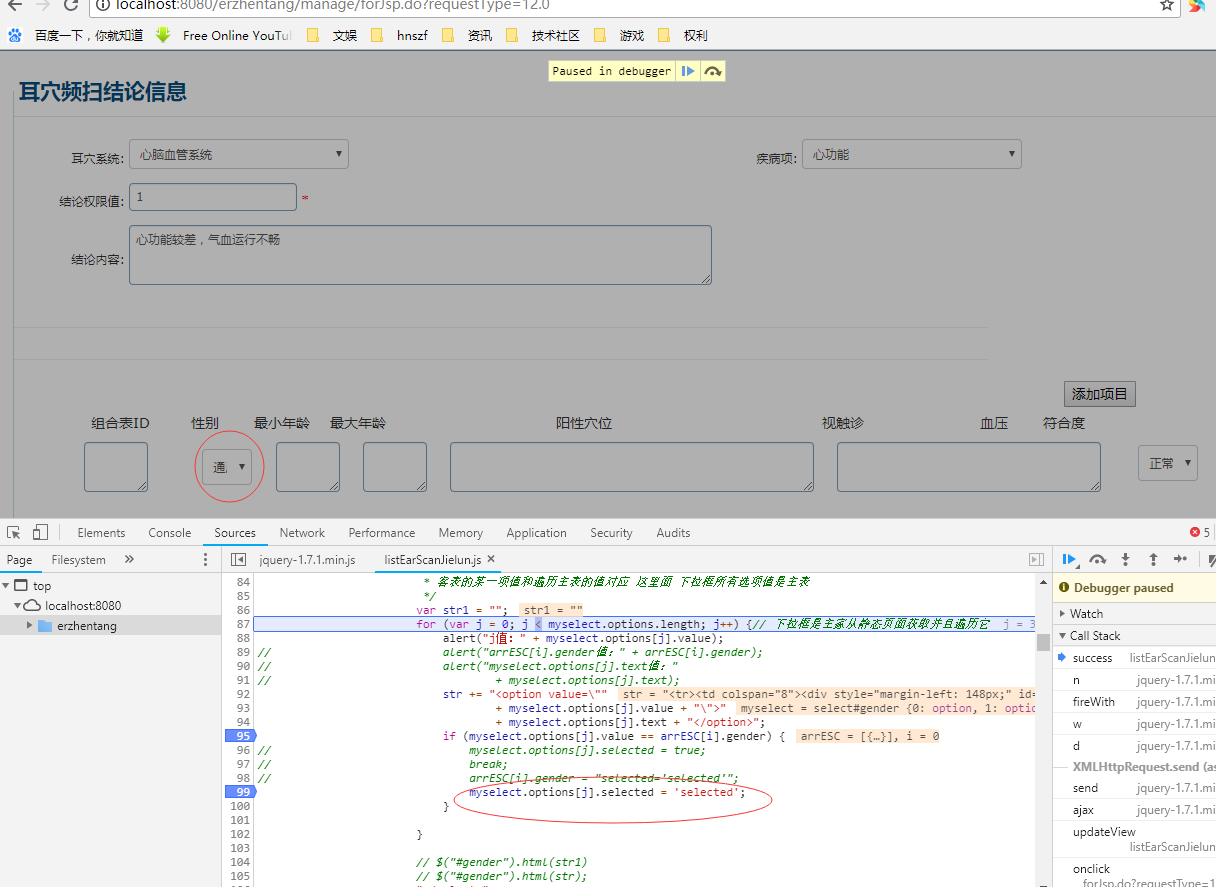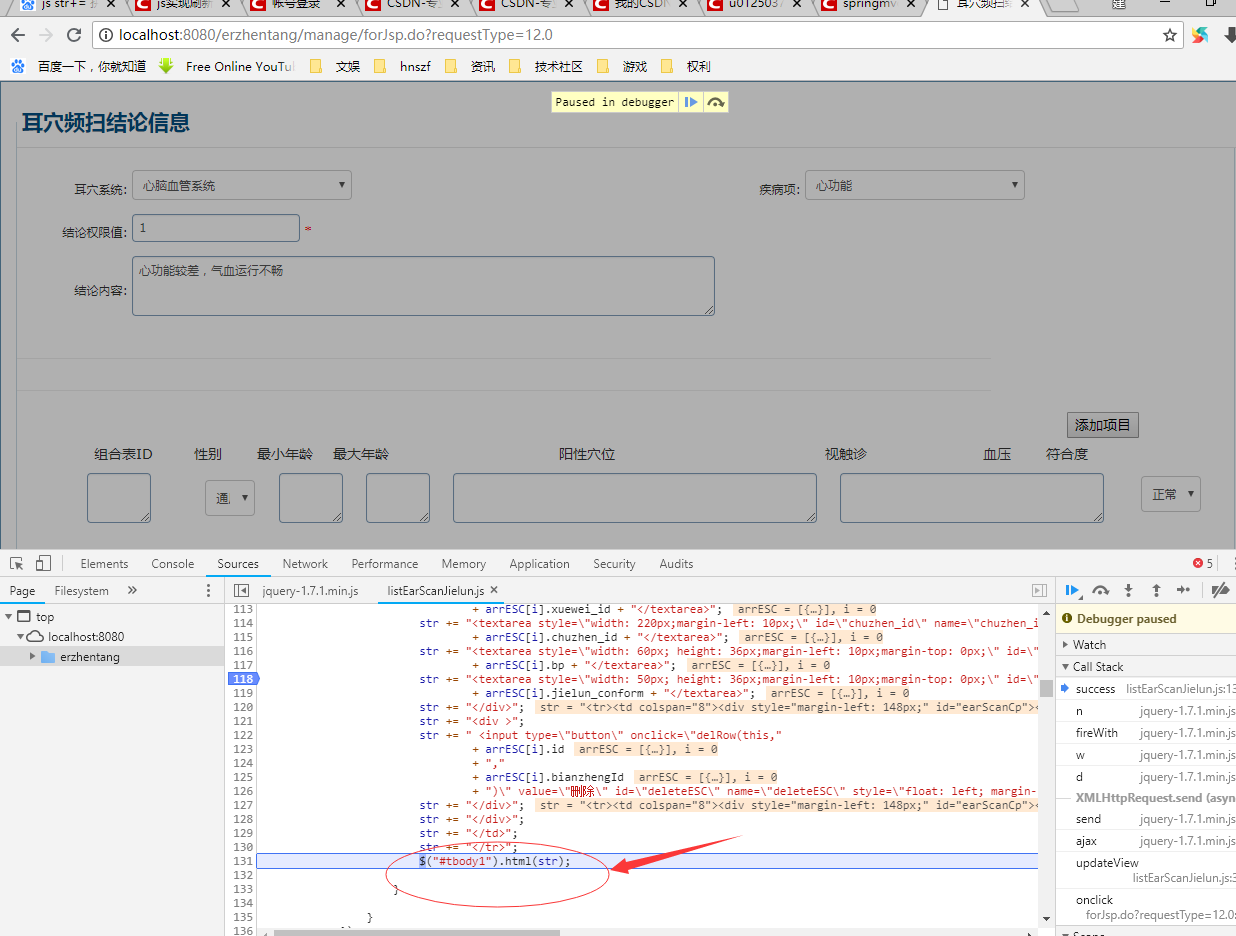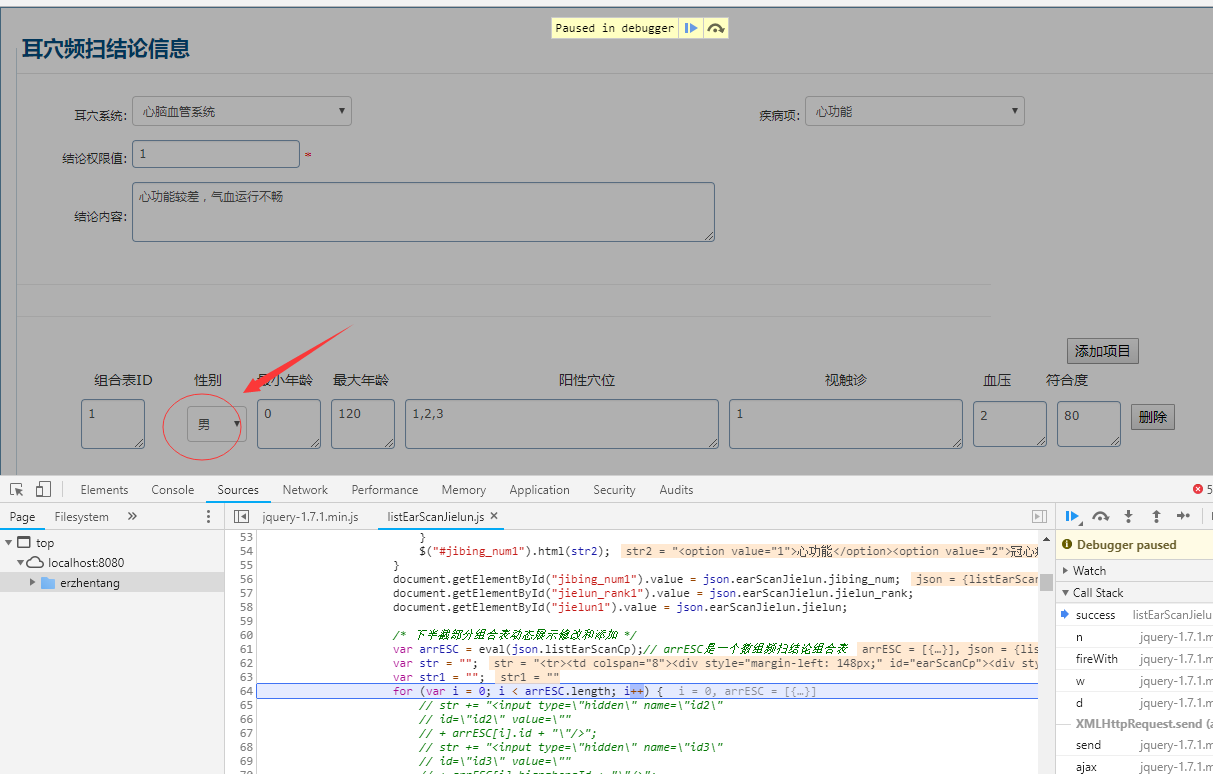js 用str+=拼接select标签 选中状态 替换jsp静态页面下拉菜单 无法展示选中状态

js部分代码

``````    /* 下半截部分组合表动态展示修改和添加 */
var arrESC = eval(json.listEarScanCp);// arrESC是一个数组频扫结论组合表
var str = "";
var str1 = "";
for (var i = 0; i < arrESC.length; i++) {
// str += "<input type=\"hidden\" name=\"id2\"
// id=\"id2\" value=\""
// + arrESC[i].id + "\"/>";
// str += "<input type=\"hidden\" name=\"id3\"
// id=\"id3\" value=\""
// + arrESC[i].bianzhengId + "\"/>";

str += "<tr>";
str += "<td colspan=\"8\">";
str += "<div style=\"margin-left: 148px;\" id=\"earScanCp\">";
str += "<div style=\"float: left;\">";
str += "<textarea style=\"width: 50px;margin-left: -86px;position: absolute;\" id=\"cp_id\" name=\"cp_id\" >"
+ arrESC[i].id + "</textarea>";
str += "<select style=\"width: 60px;height: 36px;margin-left: 20px;\" id=\"gender\" name=\"gender\" >"
var myselect = document.getElementById("gender");// 这个获取的是静态jsp页面里标签
// var index = myselect.selectedIndex;
/*
* 下拉框主要是应展示项和所有下拉选项的值判断是否对应 分清主和客 所有展示项目为主表
* 客表的某一项值和遍历主表的值对应 这里面 下拉框所有选项值是主表
*/
var str1 = "";
for (var j = 0; j < myselect.options.length; j++) {// 下拉框是主家从静态页面获取并且遍历它
//                                  + myselect.options[j].text);
str += "<option value=\""
+ myselect.options[j].value + "\">"
+ myselect.options[j].text + "</option>";
if (myselect.options[j].value == arrESC[i].gender) {
//                              myselect.options[j].selected = true;
//                              break;
//                              arrESC[i].gender = "selected='selected'";
myselect.options[j].selected = 'selected';
}

}

// \$("#gender").html(str1)
// \$("#gender").html(str);
"</select>";
arrESC[i].gender = "selected='selected'";
str += "<textarea style=\"width: 50px;margin-left: 10px;\" id=\"min_age\" name=\"min_age\" >"
+ arrESC[i].min_age + "</textarea>";
str += "<textarea style=\"width: 50px;margin-left: 10px;\" id=\"max_age\" name=\"max_age\" >"
+ arrESC[i].max_age + "</textarea>";
str += "<textarea style=\"width: 300px;margin-left: 10px;\" id=\"xuewei_id\" name=\"xuewei_id\" >"
+ arrESC[i].xuewei_id + "</textarea>";
str += "<textarea style=\"width: 220px;margin-left: 10px;\" id=\"chuzhen_id\" name=\"chuzhen_id\" >"
+ arrESC[i].chuzhen_id + "</textarea>";
str += "<textarea style=\"width: 60px; height: 36px;margin-left: 10px;margin-top: 0px;\" id=\"bp\" name=\"bp\">"
+ arrESC[i].bp + "</textarea>";
str += "<textarea style=\"width: 50px; height: 36px;margin-left: 10px;margin-top: 0px;\" id=\"jielun_conform\" name=\"jielun_conform\">"
+ arrESC[i].jielun_conform + "</textarea>";
str += "</div>";
str += "<div >";
str += " <input type=\"button\" onclick=\"delRow(this,"
+ arrESC[i].id
+ ","
+ arrESC[i].bianzhengId
+ ")\" value=\"删除\" id=\"deleteESC\" name=\"deleteESC\" style=\"float: left; margin-left:10px; margin-top: 5px;\">";
str += "</div>";
str += "</div>";
str += "</td>";
str += "</tr>";
\$("#tbody1").html(str);

}
``````

jsp页面代码

`````` <div id="bottom">
id="saveXiangmu" name="saveXiangmu"
style="float: right; margin-right: 95px;">
style="width: 100%" id="rowTable1">
<th></th>
<tr>
<td colspan="8" style="line-height: 40px;"><label
style="position:  absolute;float: left; margin-left: 75px;">组合表ID</label> <label
style="float: left; margin-left: 175px;">性别</label> <label
style="float: left; margin-left: 35px;">最小年龄</label> <label
style="float: left; margin-left: 20px;">最大年龄</label> <label
style="float: left; margin-left: 170px;">阳性穴位</label>
<label style="float: left; margin-left: 210px;">视触诊</label>
<label style="float: left; margin-left: 116px;">血压</label> <label
style="float: left; margin-left: 35px;">符合度</label></td>
</tr>
<!-- tbody里面是动态添加的内容 -->
<tbody id="tbody1">
<tr>
<td colspan="8">

<div style="margin-left: 148px;" id="earScanCp">
<div style="float: left;">
<textarea
style="width: 50px; margin-left: -80px; position: absolute;"></textarea>
<select
style="width: 50px; height: 36px; margin-left: 4%;" id="gender">
<!--  position: absolute;-->
<option value=0>男</option>
<option value=1>女</option>
<option value=2>通用</option>
</select>
<textarea style="width: 50px; margin-left: 2%;"></textarea>
<textarea
style="width: 50px; margin-left: 2%; /* margin-top: -2.5%; */"></textarea>
<textarea
style="width: 350px; margin-left: 2%; /* margin-top: -2.5%; */"></textarea>
<textarea
style="width: 250px; margin-left: 2%;/*  margin-top: -2.5%; */"></textarea>
<select
style="width: 60px; height: 36px;margin-left: 102%;margin-top: -6%;">
<option value=0>正常</option>
<option value=1>高血压</option>
<option value=2>低血压</option>
</select>
<textarea style="width: 50px; margin-left: 112%;margin-top: -6%;">50</textarea>
<!-- <input type="hidden" name="id2" id="id2" /> -->
</div>
</div>
</td>
</tr>
</tbody>
</table>
</div>
``````6个回答

``````                        var str1 = "";
for (var j = 0; j < myselect.options.length; j++) {// 下拉框是主家从静态页面获取并且遍历它
//                                  + myselect.options[j].text);
str += "<option value=\""
+ myselect.options[j].value + "\" "
+ (myselect.options[j].value == arrESC[i].gender?"selected='selected'":"") +">"
+ myselect.options[j].text + "</option>";
}

``````

Mustache.js 模板的使用

js 闭包，了解一下， 这是闭包导致的。